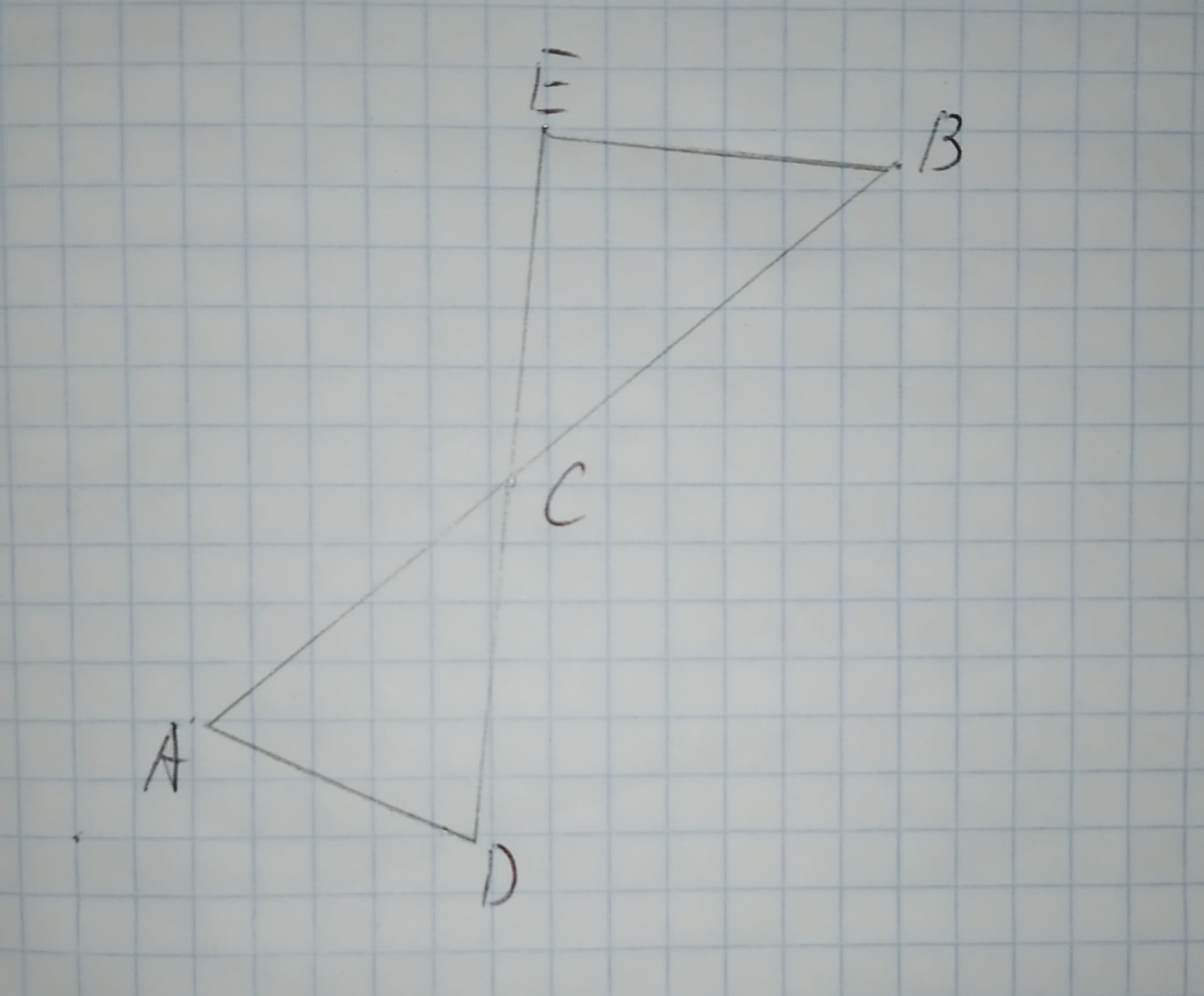Question# To check: whether the additional information in the given option would be enough to prove the given similarity. Given: The given similarity is /_ADC

Similarity
ANSWEREDTo check: whether the additional information in the given option would be enough to prove the given similarity.
Given:
The given similarity is $$\displaystyle\triangle{A}{D}{C}\sim\triangle{B}{E}{C}$$The given options are:
A.$$\displaystyle\angle{D}{A}{C}{\quad\text{and}\quad}\angle{E}{C}{B}$$ are congruent.
B.$$\displaystyle\overline{{{A}{C}}}{\quad\text{and}\quad}\overline{{{B}{C}}}$$ are congruent.
C.$$\displaystyle\overline{{{A}{D}}}{\quad\text{and}\quad}\overline{{{E}{B}}}$$ are parallel.
D.$$\displaystyle\angle{C}{E}{B}$$ is a right triangle.The given similar triangle is $$\displaystyle\triangle{A}{D}{C}\sim\triangle{B}{E}{C}$$. And this is only possible $$\displaystyle\overline{{{A}{D}}}{\quad\text{and}\quad}\overline{{{E}{B}}}$$ are parallel.
Therefore, $$\displaystyle\angle{C}{A}{D}{\quad\text{and}\quad}\angle{C}{D}{A}$$ is equal to the $$\displaystyle\angle{C}{B}{E}{\quad\text{and}\quad}\angle{C}{E}{B}$$, so that the triangle is similar by AA-similarity